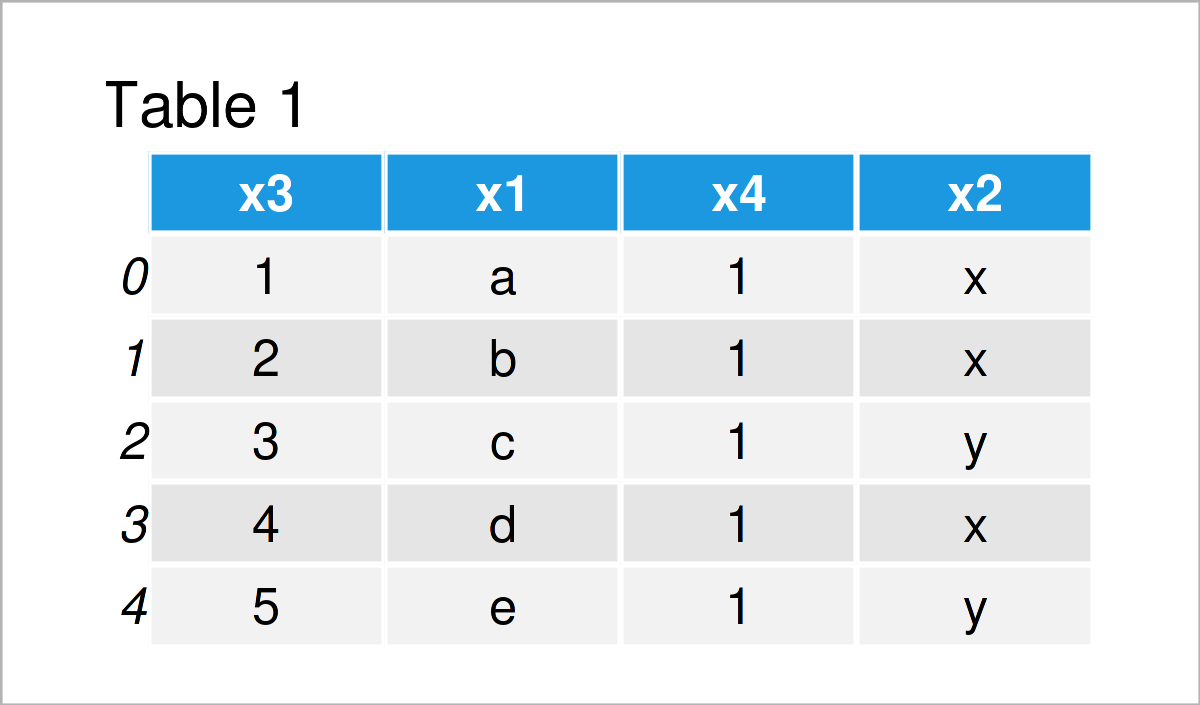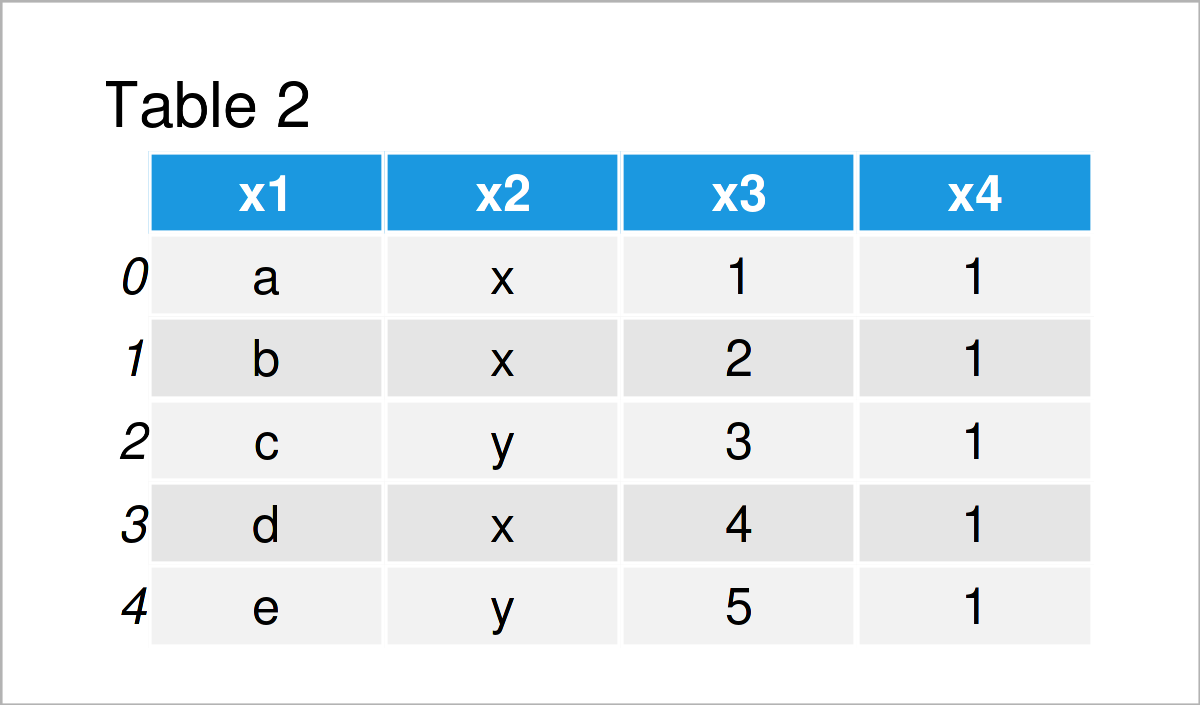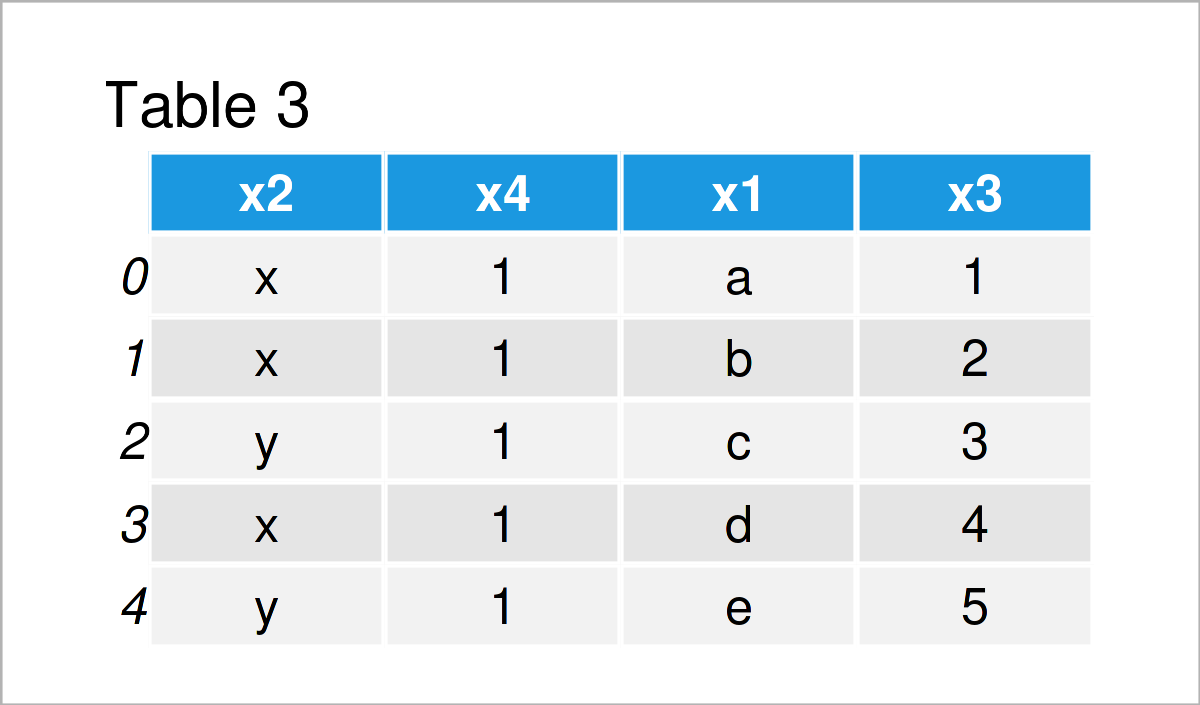# Change Order of Columns in pandas DataFrame in Python (2 Examples)

This article explains how to rearrange the variables of a pandas DataFrame in the Python programming language.

If you want to know more about these content blocks, keep reading!

## Example Data & Add-On Libraries

To be able to use the functions of the pandas library, we first need to import pandas:

`import pandas as pd                        # Load pandas library`

I’ll use the following DataFrame as basement for this Python tutorial:

```data = pd.DataFrame({'x3':range(1, 6),    # Create example DataFrame
'x1':['a', 'b', 'c', 'd', 'e'],
'x4':[1, 1, 1, 1, 1],
'x2':['x', 'x', 'y', 'x', 'y']})
print(data)                               # Print example DataFrame```Table 1 shows that the example data contains five rows and the four columns “x3”, “x1”, “x4”, and “x2”.

The following examples explain how to reorder these columns in Python!

## Example 1: Order Columns of pandas DataFrame Alphabetically

The following syntax illustrates how to sort the variables of a pandas DataFrame alphabetically by their variable name.

For this, we can use the sort_index function. Within the sort_index function, we have to specify the axis argument to be equal to 1:

```data_new1 = data.sort_index(axis=1)
print(data_new1)```After running the previous Python code the data set shown in Table 2 has been created. As you can see, the columns were sorted with alphabetical order.

## Example 2: Order Columns of pandas DataFrame Manually

Example 2 shows how to manually specify the ordering of the columns in a pandas DataFrame.

To do this, we have to specify a list containing the variable names of our DataFrame in the order that we want to set:

```data_new2 = data[['x2', 'x4', 'x1', 'x3']]
print(data_new2)```As revealed in Table 3, we have created another pandas DataFrame with user-defined ordering.

## Video, Further Resources & Summary

In case you need further info on the examples of this tutorial, I recommend having a look at the following video on my YouTube channel. In the video instruction, I’m explaining the Python programming syntax of this article in more detail.

Please accept YouTube cookies to play this video. By accepting you will be accessing content from YouTube, a service provided by an external third party.If you accept this notice, your choice will be saved and the page will refresh.

Have a look at the following video on the MyStudy YouTube channel. The speaker explains how to apply the sort_index function that we have used in Example 1 and the related sort_values function in the video:

Please accept YouTube cookies to play this video. By accepting you will be accessing content from YouTube, a service provided by an external third party.If you accept this notice, your choice will be saved and the page will refresh.

In addition, you might want to have a look at some of the other articles on this website.

At this point you should have learned how to modify and sort the column order of a pandas DataFrame in the Python programming language. Don’t hesitate to let me know in the comments section below, in case you have further questions or comments.

Subscribe to the Statistics Globe Newsletter